# 2048 Game in Python

• Difficulty Level : Easy
• Last Updated : 21 Feb, 2022

In this article we will look python code and logic to design a 2048 game you have played very often in your smartphone. If you are not familiar with the game, it is highly recommended to first play the game so that you can understand the basic functioning of it.

How to play 2048 :

1. There is a 4*4 grid which can be filled with any number. Initially two random cells are filled with 2 in it. Rest cells are empty.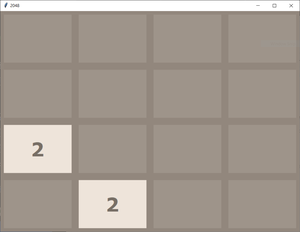2. we have to press any one of four keys to move up, down, left, or right. When we press any key, the elements of the cell move in that direction such that if any two identical numbers are contained in that particular row (in case of moving left or right) or column (in case of moving up and down) they get add up and extreme cell in that direction fill itself with that number and rest cells goes empty again.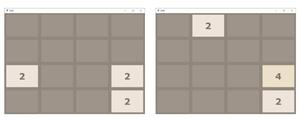3. After this grid compression any random empty cell gets itself filled with 2.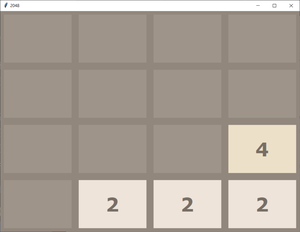4. Following the above process we have to double the elements by adding up and make 2048 in any of the cell. If we are able to do that we wins.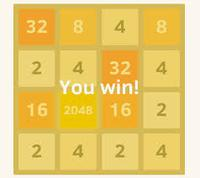5. But if during the game there is no empty cell left to be filled with a new 2, then the game goes over.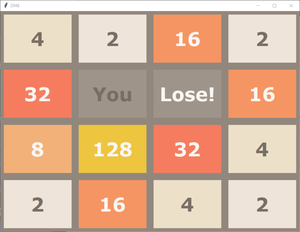In above process you can see the snapshots from graphical user interface of 2048 game. But all the logic lies in the main code. So to solely understand the logic behind it we can assume the above grid to be a 4*4 matrix ( a list with four rows and four columns). You can see below the way to take input and output without GUI for the above game.

Example :

```Commands are as follows :
'W' or 'w' : Move Up
'S' or 's' : Move Down
'A' or 'a' : Move Left
'D' or 'd' : Move Right
[0, 0, 0, 0]
[0, 0, 0, 0]
[0, 0, 0, 0]
[0, 0, 2, 0]
Press the command : a
GAME NOT OVER
[0, 0, 0, 2]
[0, 0, 0, 0]
[0, 0, 0, 0]
[2, 0, 0, 0]
Press the command : s
GAME NOT OVER
[0, 0, 0, 0]
[0, 0, 0, 0]
[0, 0, 2, 0]
[2, 0, 0, 2]
Press the command : d
GAME NOT OVER
[0, 0, 0, 0]
[0, 0, 0, 0]
[2, 0, 0, 2]
[0, 0, 0, 4]
Press the command : a
GAME NOT OVER
[0, 2, 0, 0]
[0, 0, 0, 0]
[4, 0, 0, 0]
[4, 0, 0, 0]
Press the command : s
GAME NOT OVER
[0, 0, 0, 0]
[0, 0, 0, 0]
[0, 0, 0, 0]
[8, 2, 0, 2]
.
.
.
And the series of input output will go on till we lose or win!```

Programming Approach :

1. We will design each logic function such as we are performing a left swipe then we will use it for right swipe by reversing matrix and performing left swipe.
2. Moving up can be done by taking transpose then moving left.
3. Moving down can be done by taking transpose the moving right.
4. All the logic in the program are explained in detail in the comments. Highly recommended to go through all the comments.

We have two python files below, one is 2048.py which contains main driver code and the other is logic.py which contains all functions used. logic.py should be imported in 2048.py to use these functions. just place both the files in the same folder then run 2048.py will work perfectly.

logic.py

## Python3

 `# logic.py to be``# imported in the 2048.py file` `# importing random package``# for methods to generate random``# numbers.``import` `random` `# function to initialize game / grid``# at the start``def` `start_game():` `    ``# declaring an empty list then``    ``# appending 4 list each with four``    ``# elements as 0.``    ``mat ``=``[]``    ``for` `i ``in` `range``(``4``):``        ``mat.append([``0``] ``*` `4``)` `    ``# printing controls for user``    ``print``(``"Commands are as follows : "``)``    ``print``(``"'W' or 'w' : Move Up"``)``    ``print``(``"'S' or 's' : Move Down"``)``    ``print``(``"'A' or 'a' : Move Left"``)``    ``print``(``"'D' or 'd' : Move Right"``)` `    ``# calling the function to add``    ``# a new 2 in grid after every step``    ``add_new_2(mat)``    ``return` `mat` `# function to add a new 2 in``# grid at any random empty cell``def` `add_new_2(mat):` `   ``# choosing a random index for``   ``# row and column.``    ``r ``=` `random.randint(``0``, ``3``)``    ``c ``=` `random.randint(``0``, ``3``)` `    ``# while loop will break as the``    ``# random cell chosen will be empty``    ``# (or contains zero)``    ``while``(mat[r] !``=` `0``):``        ``r ``=` `random.randint(``0``, ``3``)``        ``c ``=` `random.randint(``0``, ``3``)` `    ``# we will place a 2 at that empty``    ``# random cell.``    ``mat[r] ``=` `2` `# function to get the current``# state of game``def` `get_current_state(mat):` `    ``# if any cell contains``    ``# 2048 we have won``    ``for` `i ``in` `range``(``4``):``        ``for` `j ``in` `range``(``4``):``            ``if``(mat[i][j]``=``=` `2048``):``                ``return` `'WON'` `    ``# if we are still left with``    ``# atleast one empty cell``    ``# game is not yet over``    ``for` `i ``in` `range``(``4``):``        ``for` `j ``in` `range``(``4``):``            ``if``(mat[i][j]``=``=` `0``):``                ``return` `'GAME NOT OVER'` `    ``# or if no cell is empty now``    ``# but if after any move left, right,``    ``# up or down, if any two cells``    ``# gets merged and create an empty``    ``# cell then also game is not yet over``    ``for` `i ``in` `range``(``3``):``        ``for` `j ``in` `range``(``3``):``            ``if``(mat[i][j]``=``=` `mat[i ``+` `1``][j] ``or` `mat[i][j]``=``=` `mat[i][j ``+` `1``]):``                ``return` `'GAME NOT OVER'` `    ``for` `j ``in` `range``(``3``):``        ``if``(mat[``3``][j]``=``=` `mat[``3``][j ``+` `1``]):``            ``return` `'GAME NOT OVER'` `    ``for` `i ``in` `range``(``3``):``        ``if``(mat[i][``3``]``=``=` `mat[i ``+` `1``][``3``]):``            ``return` `'GAME NOT OVER'` `    ``# else we have lost the game``    ``return` `'LOST'` `# all the functions defined below``# are for left swap initially.` `# function to compress the grid``# after every step before and``# after merging cells.``def` `compress(mat):` `    ``# bool variable to determine``    ``# any change happened or not``    ``changed ``=` `False` `    ``# empty grid``    ``new_mat ``=` `[]` `    ``# with all cells empty``    ``for` `i ``in` `range``(``4``):``        ``new_mat.append([``0``] ``*` `4``)``        ` `    ``# here we will shift entries``    ``# of each cell to it's extreme``    ``# left row by row``    ``# loop to traverse rows``    ``for` `i ``in` `range``(``4``):``        ``pos ``=` `0` `        ``# loop to traverse each column``        ``# in respective row``        ``for` `j ``in` `range``(``4``):``            ``if``(mat[i][j] !``=` `0``):``                ` `                ``# if cell is non empty then``                ``# we will shift it's number to``                ``# previous empty cell in that row``                ``# denoted by pos variable``                ``new_mat[i][pos] ``=` `mat[i][j]``                ` `                ``if``(j !``=` `pos):``                    ``changed ``=` `True``                ``pos ``+``=` `1` `    ``# returning new compressed matrix``    ``# and the flag variable.``    ``return` `new_mat, changed` `# function to merge the cells``# in matrix after compressing``def` `merge(mat):``    ` `    ``changed ``=` `False``    ` `    ``for` `i ``in` `range``(``4``):``        ``for` `j ``in` `range``(``3``):` `            ``# if current cell has same value as``            ``# next cell in the row and they``            ``# are non empty then``            ``if``(mat[i][j] ``=``=` `mat[i][j ``+` `1``] ``and` `mat[i][j] !``=` `0``):` `                ``# double current cell value and``                ``# empty the next cell``                ``mat[i][j] ``=` `mat[i][j] ``*` `2``                ``mat[i][j ``+` `1``] ``=` `0` `                ``# make bool variable True indicating``                ``# the new grid after merging is``                ``# different.``                ``changed ``=` `True` `    ``return` `mat, changed` `# function to reverse the matrix``# means reversing the content of``# each row (reversing the sequence)``def` `reverse(mat):``    ``new_mat ``=``[]``    ``for` `i ``in` `range``(``4``):``        ``new_mat.append([])``        ``for` `j ``in` `range``(``4``):``            ``new_mat[i].append(mat[i][``3` `-` `j])``    ``return` `new_mat` `# function to get the transpose``# of matrix means interchanging``# rows and column``def` `transpose(mat):``    ``new_mat ``=` `[]``    ``for` `i ``in` `range``(``4``):``        ``new_mat.append([])``        ``for` `j ``in` `range``(``4``):``            ``new_mat[i].append(mat[j][i])``    ``return` `new_mat` `# function to update the matrix``# if we move / swipe left``def` `move_left(grid):` `    ``# first compress the grid``    ``new_grid, changed1 ``=` `compress(grid)` `    ``# then merge the cells.``    ``new_grid, changed2 ``=` `merge(new_grid)``    ` `    ``changed ``=` `changed1 ``or` `changed2` `    ``# again compress after merging.``    ``new_grid, temp ``=` `compress(new_grid)` `    ``# return new matrix and bool changed``    ``# telling whether the grid is same``    ``# or different``    ``return` `new_grid, changed` `# function to update the matrix``# if we move / swipe right``def` `move_right(grid):` `    ``# to move right we just reverse``    ``# the matrix``    ``new_grid ``=` `reverse(grid)` `    ``# then move left``    ``new_grid, changed ``=` `move_left(new_grid)` `    ``# then again reverse matrix will``    ``# give us desired result``    ``new_grid ``=` `reverse(new_grid)``    ``return` `new_grid, changed` `# function to update the matrix``# if we move / swipe up``def` `move_up(grid):` `    ``# to move up we just take``    ``# transpose of matrix``    ``new_grid ``=` `transpose(grid)` `    ``# then move left (calling all``    ``# included functions) then``    ``new_grid, changed ``=` `move_left(new_grid)` `    ``# again take transpose will give``    ``# desired results``    ``new_grid ``=` `transpose(new_grid)``    ``return` `new_grid, changed` `# function to update the matrix``# if we move / swipe down``def` `move_down(grid):` `    ``# to move down we take transpose``    ``new_grid ``=` `transpose(grid)` `    ``# move right and then again``    ``new_grid, changed ``=` `move_right(new_grid)` `    ``# take transpose will give desired``    ``# results.``    ``new_grid ``=` `transpose(new_grid)``    ``return` `new_grid, changed` `# this file only contains all the logic``# functions to be called in main function``# present in the other file`

## Python3

 `# logic.py to be``# imported in the 2048.py file` `# importing random package``# for methods to generate random``# numbers.``import` `random` `# function to initialize game / grid``# at the start``def` `start_game():` `    ``# declaring an empty list then``    ``# appending 4 list each with four``    ``# elements as 0.``    ``mat ``=``[]``    ``for` `i ``in` `range``(``4``):``        ``mat.append([``0``] ``*` `4``)` `    ``# printing controls for user``    ``print``(``"Commands are as follows : "``)``    ``print``(``"'W' or 'w' : Move Up"``)``    ``print``(``"'S' or 's' : Move Down"``)``    ``print``(``"'A' or 'a' : Move Left"``)``    ``print``(``"'D' or 'd' : Move Right"``)` `    ``# calling the function to add``    ``# a new 2 in grid after every step``    ``add_new_2(mat)``    ``return` `mat` `# function to add a new 2 in``# grid at any random empty cell``def` `add_new_2(mat):` `   ``# choosing a random index for``   ``# row and column.``    ``r ``=` `random.randint(``0``, ``3``)``    ``c ``=` `random.randint(``0``, ``3``)` `    ``# while loop will break as the``    ``# random cell chosen will be empty``    ``# (or contains zero)``    ``while``(mat[r] !``=` `0``):``        ``r ``=` `random.randint(``0``, ``3``)``        ``c ``=` `random.randint(``0``, ``3``)` `    ``# we will place a 2 at that empty``    ``# random cell.``    ``mat[r] ``=` `2` `# function to get the current``# state of game``def` `get_current_state(mat):` `    ``# if any cell contains``    ``# 2048 we have won``    ``for` `i ``in` `range``(``4``):``        ``for` `j ``in` `range``(``4``):``            ``if``(mat[i][j]``=``=` `2048``):``                ``return` `'WON'` `    ``# if we are still left with``    ``# atleast one empty cell``    ``# game is not yet over``    ``for` `i ``in` `range``(``4``):``        ``for` `j ``in` `range``(``4``):``            ``if``(mat[i][j]``=``=` `0``):``                ``return` `'GAME NOT OVER'` `    ``# or if no cell is empty now``    ``# but if after any move left, right,``    ``# up or down, if any two cells``    ``# gets merged and create an empty``    ``# cell then also game is not yet over``    ``for` `i ``in` `range``(``3``):``        ``for` `j ``in` `range``(``3``):``            ``if``(mat[i][j]``=``=` `mat[i ``+` `1``][j] ``or` `mat[i][j]``=``=` `mat[i][j ``+` `1``]):``                ``return` `'GAME NOT OVER'` `    ``for` `j ``in` `range``(``3``):``        ``if``(mat[``3``][j]``=``=` `mat[``3``][j ``+` `1``]):``            ``return` `'GAME NOT OVER'` `    ``for` `i ``in` `range``(``3``):``        ``if``(mat[i][``3``]``=``=` `mat[i ``+` `1``][``3``]):``            ``return` `'GAME NOT OVER'` `    ``# else we have lost the game``    ``return` `'LOST'` `# all the functions defined below``# are for left swap initially.` `# function to compress the grid``# after every step before and``# after merging cells.``def` `compress(mat):` `    ``# bool variable to determine``    ``# any change happened or not``    ``changed ``=` `False` `    ``# empty grid``    ``new_mat ``=` `[]` `    ``# with all cells empty``    ``for` `i ``in` `range``(``4``):``        ``new_mat.append([``0``] ``*` `4``)``        ` `    ``# here we will shift entries``    ``# of each cell to it's extreme``    ``# left row by row``    ``# loop to traverse rows``    ``for` `i ``in` `range``(``4``):``        ``pos ``=` `0` `        ``# loop to traverse each column``        ``# in respective row``        ``for` `j ``in` `range``(``4``):``            ``if``(mat[i][j] !``=` `0``):``                ` `                ``# if cell is non empty then``                ``# we will shift it's number to``                ``# previous empty cell in that row``                ``# denoted by pos variable``                ``new_mat[i][pos] ``=` `mat[i][j]``                ` `                ``if``(j !``=` `pos):``                    ``changed ``=` `True``                ``pos ``+``=` `1` `    ``# returning new compressed matrix``    ``# and the flag variable.``    ``return` `new_mat, changed` `# function to merge the cells``# in matrix after compressing``def` `merge(mat):``    ` `    ``changed ``=` `False``    ` `    ``for` `i ``in` `range``(``4``):``        ``for` `j ``in` `range``(``3``):` `            ``# if current cell has same value as``            ``# next cell in the row and they``            ``# are non empty then``            ``if``(mat[i][j] ``=``=` `mat[i][j ``+` `1``] ``and` `mat[i][j] !``=` `0``):` `                ``# double current cell value and``                ``# empty the next cell``                ``mat[i][j] ``=` `mat[i][j] ``*` `2``                ``mat[i][j ``+` `1``] ``=` `0` `                ``# make bool variable True indicating``                ``# the new grid after merging is``                ``# different.``                ``changed ``=` `True` `    ``return` `mat, changed` `# function to reverse the matrix``# means reversing the content of``# each row (reversing the sequence)``def` `reverse(mat):``    ``new_mat ``=``[]``    ``for` `i ``in` `range``(``4``):``        ``new_mat.append([])``        ``for` `j ``in` `range``(``4``):``            ``new_mat[i].append(mat[i][``3` `-` `j])``    ``return` `new_mat` `# function to get the transpose``# of matrix means interchanging``# rows and column``def` `transpose(mat):``    ``new_mat ``=` `[]``    ``for` `i ``in` `range``(``4``):``        ``new_mat.append([])``        ``for` `j ``in` `range``(``4``):``            ``new_mat[i].append(mat[j][i])``    ``return` `new_mat` `# function to update the matrix``# if we move / swipe left``def` `move_left(grid):` `    ``# first compress the grid``    ``new_grid, changed1 ``=` `compress(grid)` `    ``# then merge the cells.``    ``new_grid, changed2 ``=` `merge(new_grid)``    ` `    ``changed ``=` `changed1 ``or` `changed2` `    ``# again compress after merging.``    ``new_grid, temp ``=` `compress(new_grid)` `    ``# return new matrix and bool changed``    ``# telling whether the grid is same``    ``# or different``    ``return` `new_grid, changed` `# function to update the matrix``# if we move / swipe right``def` `move_right(grid):` `    ``# to move right we just reverse``    ``# the matrix``    ``new_grid ``=` `reverse(grid)` `    ``# then move left``    ``new_grid, changed ``=` `move_left(new_grid)` `    ``# then again reverse matrix will``    ``# give us desired result``    ``new_grid ``=` `reverse(new_grid)``    ``return` `new_grid, changed` `# function to update the matrix``# if we move / swipe up``def` `move_up(grid):` `    ``# to move up we just take``    ``# transpose of matrix``    ``new_grid ``=` `transpose(grid)` `    ``# then move left (calling all``    ``# included functions) then``    ``new_grid, changed ``=` `move_left(new_grid)` `    ``# again take transpose will give``    ``# desired results``    ``new_grid ``=` `transpose(new_grid)``    ``return` `new_grid, changed` `# function to update the matrix``# if we move / swipe down``def` `move_down(grid):` `    ``# to move down we take transpose``    ``new_grid ``=` `transpose(grid)` `    ``# move right and then again``    ``new_grid, changed ``=` `move_right(new_grid)` `    ``# take transpose will give desired``    ``# results.``    ``new_grid ``=` `transpose(new_grid)``    ``return` `new_grid, changed` `# this file only contains all the logic``# functions to be called in main function``# present in the other file`

2048.py

## Python3

 `# 2048.py` `# importing the logic.py file``# where we have written all the``# logic functions used.``import` `logic` `# Driver code``if` `__name__ ``=``=` `'__main__'``:``    ` `# calling start_game function``# to initialize the matrix``    ``mat ``=` `logic.start_game()` `while``(``True``):` `    ``# taking the user input``    ``# for next step``    ``x ``=` `input``(``"Press the command : "``)` `    ``# we have to move up``    ``if``(x ``=``=` `'W'` `or` `x ``=``=` `'w'``):` `        ``# call the move_up function``        ``mat, flag ``=` `logic.move_up(mat)` `        ``# get the current state and print it``        ``status ``=` `logic.get_current_state(mat)``        ``print``(status)` `        ``# if game not ove then continue``        ``# and add a new two``        ``if``(status ``=``=` `'GAME NOT OVER'``):``            ``logic.add_new_2(mat)` `        ``# else break the loop``        ``else``:``            ``break` `    ``# the above process will be followed``    ``# in case of each type of move``    ``# below` `    ``# to move down``    ``elif``(x ``=``=` `'S'` `or` `x ``=``=` `'s'``):``        ``mat, flag ``=` `logic.move_down(mat)``        ``status ``=` `logic.get_current_state(mat)``        ``print``(status)``        ``if``(status ``=``=` `'GAME NOT OVER'``):``            ``logic.add_new_2(mat)``        ``else``:``            ``break` `    ``# to move left``    ``elif``(x ``=``=` `'A'` `or` `x ``=``=` `'a'``):``        ``mat, flag ``=` `logic.move_left(mat)``        ``status ``=` `logic.get_current_state(mat)``        ``print``(status)``        ``if``(status ``=``=` `'GAME NOT OVER'``):``            ``logic.add_new_2(mat)``        ``else``:``            ``break` `    ``# to move right``    ``elif``(x ``=``=` `'D'` `or` `x ``=``=` `'d'``):``        ``mat, flag ``=` `logic.move_right(mat)``        ``status ``=` `logic.get_current_state(mat)``        ``print``(status)``        ``if``(status ``=``=` `'GAME NOT OVER'``):``            ``logic.add_new_2(mat)``        ``else``:``            ``break``    ``else``:``        ``print``(``"Invalid Key Pressed"``)` `    ``# print the matrix after each``    ``# move.``    ``print``(mat)`

Output :

My Personal Notes arrow_drop_up